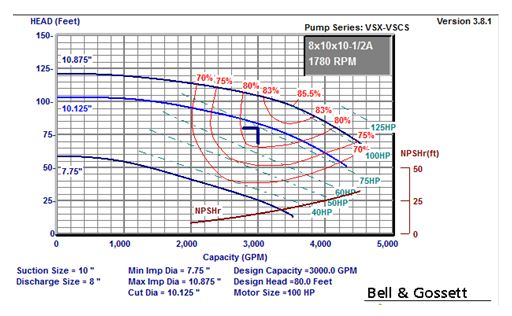# It’s all about Pressure – Cooling Tower Pumps and Piping

Norm Hall
/
April 30, 2012

Last week the R. L. Deppmann Monday Morning Minute left you with a multiplier of 1.3, 1.5, or 2.0 to use with the pump NPSHR. Let’s look at an example this week using the suction energy formula we introduced last week and add one more margin to the formula.

Let’s start with the additional margin. Tower water has a great deal of air entrained and that air can play havoc with the gauge pressure at the pump suction. How often have you witnessed the bouncing gauge in this situation? This is the reason I add 5 extra feet to the NPSHR as a safety factor. You may choose to add this or not, but I always start by adding it in. Now let’s look at an example. I suggest you have the last Monday Morning minute handy.Example: Cooling Tower Pump for 3000 GPM at 80 feet using a B&G series VSX-VSCS-8x10x10.5A   D = suction size = 10″ n = speed = 1780 Q = 3514 GPM at BEP and max. Impeller/2 = 1757 because this is a double suction impeller. NPSH = 20 at BEP and max impeller. So from the last MMM: Suction Energy = D x n x (RPM x Q.5 /NPSHR.75) X10-6 So Suction Energy = 10 x 1780 x (1780 x (1757) .5 / (20) .75) x (.000001)≈ 140 which is a low energy pump.

Now, from the chart published in the last Monday Morning Minute, the multiplier to use with published NPSHR will be 1.3 and the recommended safety factor from statements above will be 5 feet. The NPSHR at 3000 GPM is 15 feet absolute from the curve above. We will want our available NPSH to be [[15 x 1.3 = 19.5 + 5 = 24.5]] feet absolute or greater.